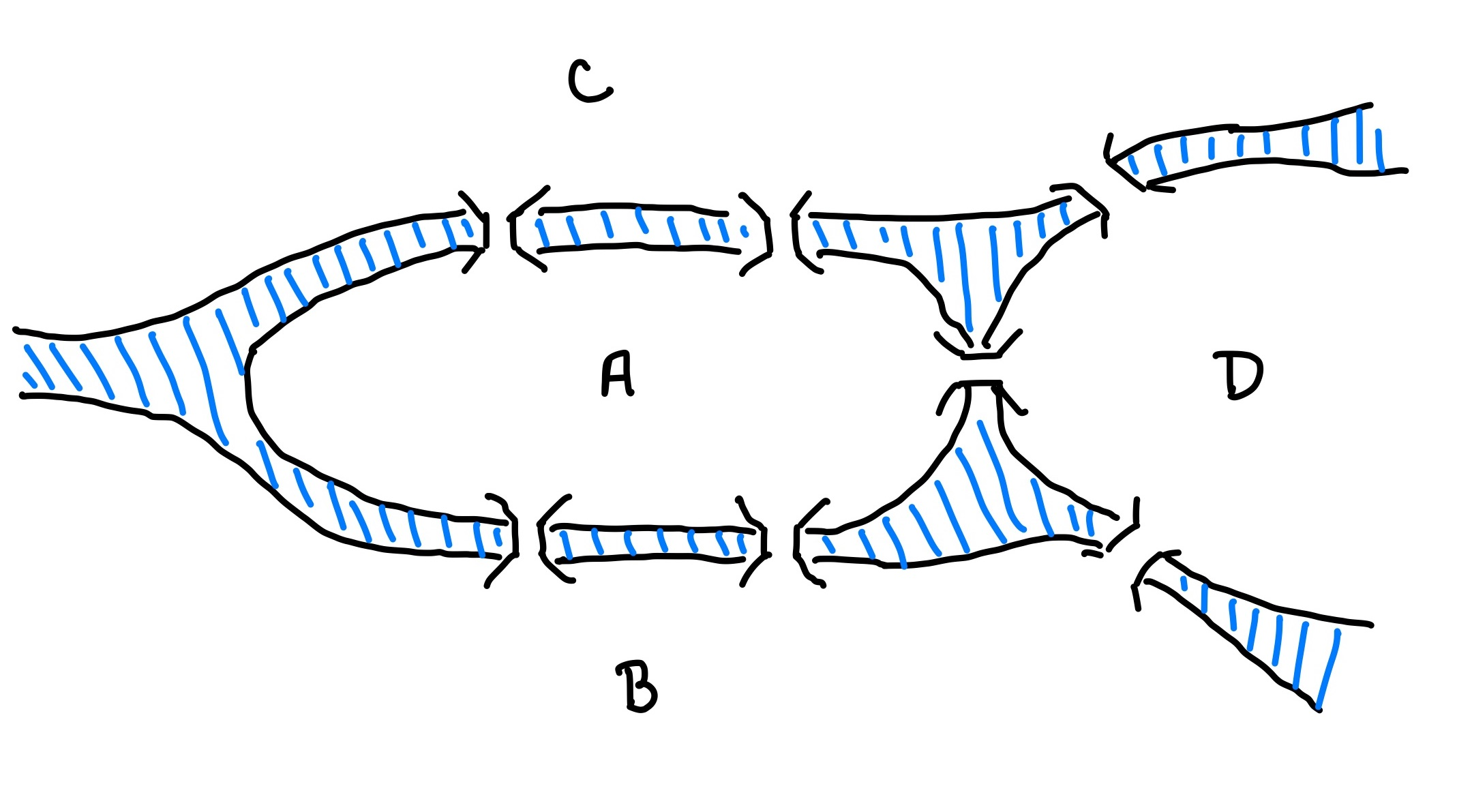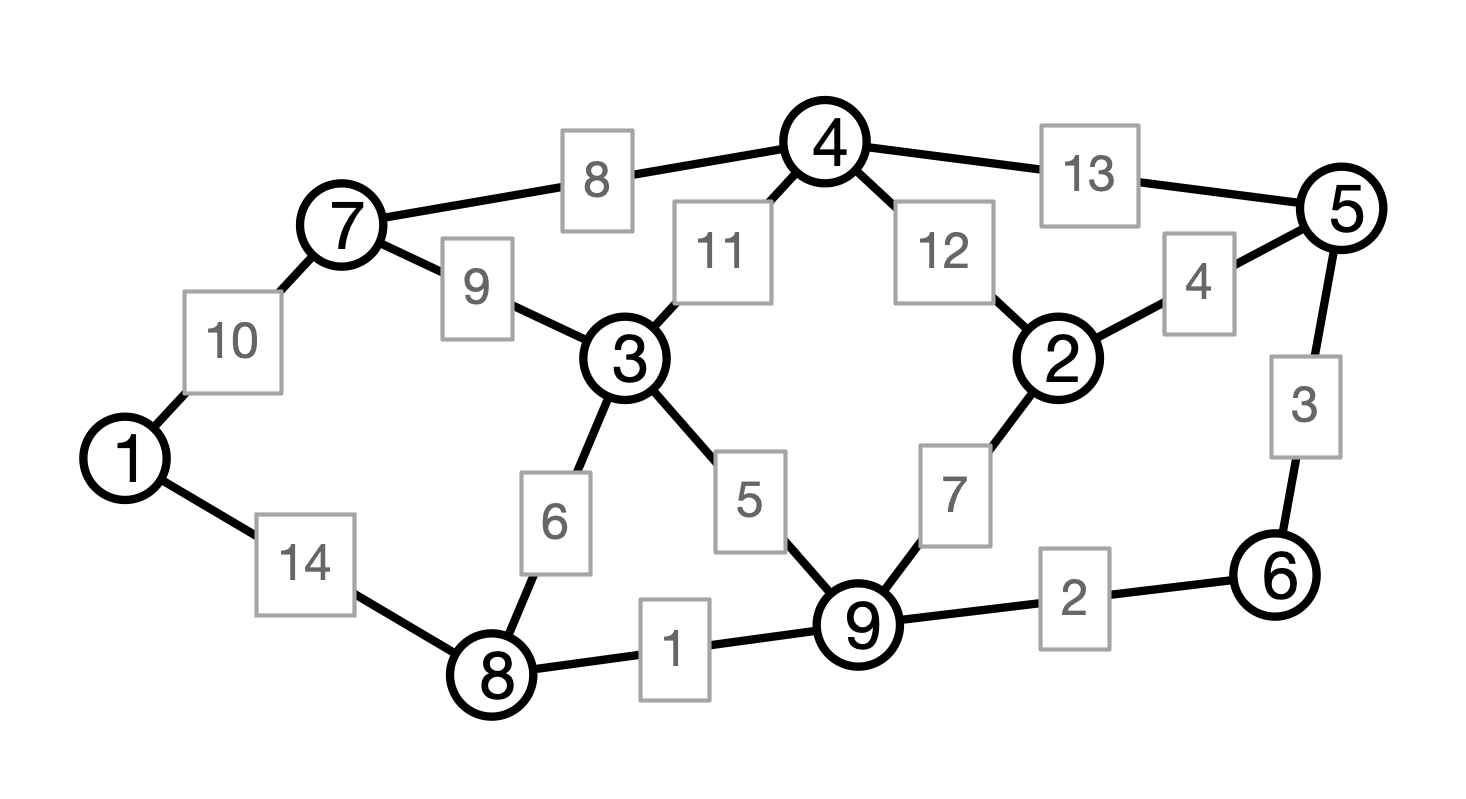# Lecture 18: Minimum Spanning Trees

### COSC 311 Algorithms, Fall 2022

$\def\compare{ {\mathrm{compare}} } \def\swap{ {\mathrm{swap}} } \def\sort{ {\mathrm{sort}} } \def\insert{ {\mathrm{insert}} } \def\true{ {\mathrm{true}} } \def\false{ {\mathrm{false}} } \def\BubbleSort{ {\mathrm{BubbleSort}} } \def\SelectionSort{ {\mathrm{SelectionSort}} } \def\Merge{ {\mathrm{Merge}} } \def\MergeSort{ {\mathrm{MergeSort}} } \def\QuickSort{ {\mathrm{QuickSort}} } \def\Split{ {\mathrm{Split}} } \def\Multiply{ {\mathrm{Multiply}} } \def\Add{ {\mathrm{Add}} } \def\cur{ {\mathrm{cur}} } \def\gets{ {\leftarrow} }$

## Announcements

1. Midterms
• pick up in class Wednesday or OH on Thursday
2. No class on Monday 10/24

## Overview

1. Minimum Spanning Tree Problem
2. Prim’s Algorithm

## Last Time: Dijkstra’s Algorithm

Single Source Shortest Paths

• Input
• graph $G = (V, E)$
• edge weights/lengths $w$
• starting vertex $u$
• Output
• (weighted) distance $d[v] = d_w(u, v)$ for every vertex $v$

Dijkstra’s Algorithm:

• maintain set $S$ of finalized nodes
• greedily choose $v \in V - S$ with minimum $d[v]$
• finalize $v$
• update $d[x]$ for each neighbor $x$ of $v$

## DPW in Königsberg

Winter is coming to Königsberg!

• Must prepare the bridges for ice
• Each bridge has an associated cost to de-ice
• Königsberg doesn’t have the budget to de-ice all bridges
• For public safety, must ensure that all landmasses are reachable from each other

Question. How to find the cheapest set of bridges to de-ice that maintain connectivity?

## Picture## Graph Problem

Input:

• a weighted graph $G = (V, E)$ with edge weights $w$

Output:

• a set $F$ of edges in $E$ such that

1. $(V, F)$ is connected
2. sum of weights of edges in $F$ is minimal among all connected sub-graphs of $G$

The graph $T = (V, F)$ is called a minimum spanning tree of $G$

## Example## Trees

Recall. A tree $T$ is a graph that:

1. is connected, and
2. contains no cycles

## MST Problem

Input:

• a weighted graph $G = (V, E)$ with edge weights $w$

Output:

• a set $F$ of edges in $E$ such that

1. $(V, F)$ is connected
2. sum of weights of edges in $F$ is minimal among all connected sub-graphs of $G$

Question. Why must $T = (V, F)$ be a tree?

## A Claim

Claim. Suppose $T$ is an MST for a weighted graph $G$. Then $T$ is a tree.

• Suppose $T$ is not a tree
• By definition of MST, $T$ is connected
• So $T$ must contain a cycle $C$
• Removing any edge $e$ from $C$ results in a smaller spanning “tree”
• $\implies T$ was not minimal, a contradiction

## An Idea

Grow a tree outward from a seed vertex:

• start with $u$
• repeat until we get a spanning tree:
• pick a new edge $e$ to add to our tree

Question. How should we pick the “next” edge?

## Tree Growing Example## Question

What information do we need to maintain in order to implement this procedure?

## Prim’s Algorithm

  PrimMST(V, E):
initialize set S = {v} with v arbitrary
initialize set F = {} of MST edges, priority queue Q
for each neighbor x of v
add (v, x) to Q with priority w(v, x)
while Q is not empty
(u, v) <- removeMin(Q)
if S doesn't contain v
for each neighbor x of v
add (v, x) to Q with priority w(v, x)
return (S, F)


## Prim Illustration## Question

Why is graph returned by Prim a tree?

  PrimMST(V, E):
initialize set S = {v} with v arbitrary
initialize set F = {} of MST edges, priority queue Q
for each neighbor x of v
add (v, x) to Q with priority w(v, x)
while Q is not empty
(u, v) <- removeMin(Q)
if S doesn't contain v
for each neighbor x of v
add (v, x) to Q with priority w(v, x)
return (S, F)


## Another Question

Why is graph returned by Prim a MST?

• assume all edge weights are distinct

Must show. Every edge added to $F$ is in an MST.

## Cuts in Graphs

Definition. Let $G = (V, E)$ be a graph. A cut in $G$ is a partition of $V$ into two (non-empty) subsets $U$ and $V - U$.## Cuts and MSTs

Cut Claim. Suppose:

• $G = (V, E)$ is a weighted graph (with distinct edge weights)
• $U, V - U$ a cut in $G$
• $T = (V, F)$ an MST
• $e = (u, v)$ is the minimum weight edge that crosses the cut
• $u \in U$ and $v \in V - U$

Then:

• $T$ contains the edge $e$

## Cut Claim Illustration## Prim, Again

  PrimMST(V, E):
initialize set S = {v} with v arbitrary
initialize set F = {} of MST edges, priority queue Q
for each neighbor x of v
add (v, x) to Q with priority w(v, x)
while Q is not empty
(u, v) <- removeMin(Q)
if S doesn't contain v
for each neighbor x of v
add (v, x) to Q with priority w(v, x)
return (S, F)


## Prim Correctness I

Cut claim $\implies$ Prim produces an MST.

Why?

Consider $k$th edge $e_k$ added by Prim

• $S_k =$ contents of $S$ before edge added

What can we say about the cut $S_k, V - S_k$?

## Prim Correctness II

First Conclusion. Every edge $e$ added by Prim’s algorithm is in the MST.

Still to show. All MST edges are added.

Why is this so?

• Suffices to argue that Prim produces a spanning tree
• Set of edges found by Prim form a tree
• All vertices of $V$ are in tree (if $G$ is connected)

Conclusion. Prim’s algorithm produces an MST.

## Prim Running Time?

  PrimMST(V, E):
initialize set S = {v} with v arbitrary
initialize set F = {} of MST edges, priority queue Q
for each neighbor x of v
add (v, x) to Q with priority w(v, x)
while Q is not empty
(u, v) <- removeMin(Q)
if S doesn't contain v
for each neighbor x of v
add (v, x) to Q with priority w(v, x)
return (S, F)


## Conclusion

Prim’s algorithm:

• computes an MST in $G$
• if efficient priority queue is used, running time is $O(m \log n)$

Prim’s algorithm is greedy

• to grow a tree, always add the lightest outgoing edge

## Next Time

Another greedy MST algorithm!